## Saturday, May 27, 2006

### My Growing Post Due Monday May 29th

Question 1: What is the difference between an algebraic expression and an equation (hint: One contains this and the other doesn't)? Give an example of both an expression and an equation.

In an algebraic expression you get to choose the value of the variable.
In an algebraic equation the value of the variable is given.
Algebraic Expression: 9N+4
Algebraic Equation: 9N+4=22

Question 2: What is a variable and why do we use one in algebra?

A variable is a letter that replaces a number. We use a variable in algebra because algebra is about solving the uknown and the variable is the unknown thing we have to solve.

Question 3: Solve for N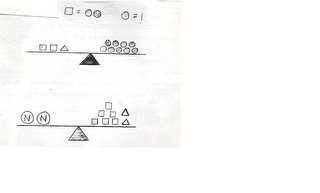To solve the second question, you need to figure out what a triangle equals on the first scale.
First, I took away four circles (each square equals two circles), which left me with five circles. Since there were five circles left, the triangle equals five circles.
To solve the second question, you need to figure out the value of the two Ns.
First, I added the squares and triangles together. The total of the squares and triangles, together, was 22 circles. Then I divided the 22 circles by 2. The answer was 11. So each N equals 11 circles.

Question 4: Solve the following equations. Show all of the steps that are needed.

(a) 3n+4n+7=2n+12

7n+7=2n+12

7n-2n(+7-7)=2n-2n(+12-7)

5n=5

n=1

(b) 8n-(4+9)=11

8n-13=11

+13 +13

8n=24

n=24/8

n=3

(c) (8-3)n+7n+8=4n+40

5n+7n+8 =4n+40

12n+8=4n+40

12n(+8-8)=4n(+40-8)

12n-4n=(4n-4n)+32

8n=32

n=4

Question 5: For this question you need to create a T-Chart and a graph to plot your data from this question onto.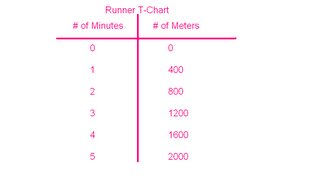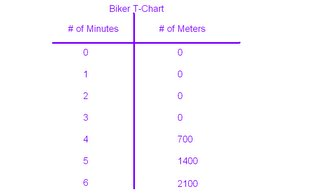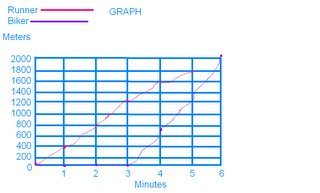You are having a race against your friend, except you are on foot and he is on his bike. Your both know that if you are on a bike you will be faster so your friend gives you a head start of 3 minutes. If you can run 400m per minute, and your friend can bike 700m per minute, who will be the first to make it to the finish line 2000m away?

The runner will cross the finish line first in 5 minutes. The biker will cross the finish line second in 6 minutes.

Kendra Valentonis 7-43

## Wednesday, May 24, 2006

### Janna's Growing Post

Quetsion #1: Solve all 3 parts of the problem below.

Jack collects baseball cards. Jack has 180 baseball cards in his collection. At the end of each month jack buys 25 baseball cards to add to his collection.

A)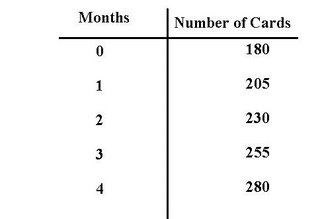B) 180 + 25( j ) = 280

C) Jack will have 1380 when he turns 12.

Question #2:

A) Jackie should order 2 pizzas . Because if each peron eats 3 pieces of pizza and there are 6 slices in a pizza. That means 2 people can finish 1 pizza. Since there are 4 people, that means they can finish 2 pizzas.

B) Jackie needs to order one more pizza. Because 2 people can finish 1 pizza.

Questions #3:

A) Cathy will have \$200.00 dollars in her saving account. I got the answer by using her formula of 20+15(12). The first thing i did was I mulitplied 12 by 15 to get 180 then i added her \$20.00 dollars to get \$200.00 dollars in total.

B)

25 + 15A = savings account
Then Cathy would have a total of \$205.00 dollars for that 1 year

Question #4:

a) 3n + 4n + 7 = 2n + 12

3n+4n+7=2n+12

3(1)+4(1)+7=2(1)+12

3+4+7=14

N= 1

b) 8n – (4 + 9) = 11

8n-(4+9)=11

8(3)-(4+9)=11

24-13=11

N= 3

c) (8 – 3 )n + 7n + 8 = 4n + 40

(8-3)n+7n+8=4n+40

5n+7n+8=4n+40

5(4)+7(4)+8=4(4)+40

20+28+8=56

N= 4

## Tuesday, May 23, 2006

### Growing Post

Question 1:

A) Create a T-Chart showing how many baseball cards Jack has at the end of the next 4 months.

B) Create an algebraic formula based upon the problem above.
180 + 25T = Amount of baseball cards
T = The Month(s)

C) If Jack just turned 8 years old this month and Jack continues to buy the same amount of baseball cards each month, how many baseball cards will jack have when he turns 12?
Jack will have 1380 card by the time he's twelve.
Forty-eight months until Jack is twelve. 48 X 25 = 1200
1200 + 180= 1380

Formula: 180 + 25T= 1380

(T = 48)

Question 2: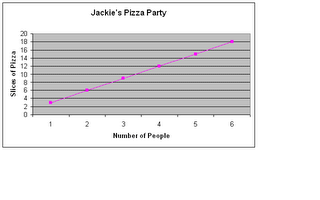A) If Jackie thinks that there will be four people at the party (counting herself) how many pizza’s should she order?
If Jackie has three friends she will need to have at least two pizzas so she can supply herself.
12 slices = 2 pizzas
12 slices = 4 people

B) If two more people show up at the party how many more pizza’s does she need to order?
If more people show up she will have to order three pizzas, since one pizza supports two people.

Question 3:

A) Calculate how much money Kathy will have in one year.
First multiply the months by the amount of money she recieves; 15 X 12 = 180
Add 20 to the sum; 200 + 20 = 200

B) Calculate how much money Kathy will have in one year.
This answer is quite simple. All you do is change the 20 in Kathy's formula, and change it to 25.
25 + 15n = Savings
If that was what Kathy had in her account, then her savings would be \$205.

Question Four:

A) 3N + 4N + 7 = 12 + 2N
3(1) + 4(1) + 7 = 2(1) = 12
3 + 4 + 7 = 14
N = 1

B) 8N - ( 4 + 9) = 11
8N - (4 + 9) = 11
8(3) - (4 + 9) = 11
24 - 13 = 11
N = 3

C) (8 - 3)N + 7N + 8 = 4N + 40
5N + 7N + 8 = 4N + 40
5(4) + 7(4) + 8 = 4(4) + 40
20 + 28 + 8 = 56
N = 4

### THE GROWING POST!!!!!!!!!!!!!!!!!!!!!!

Mark you received 15/17 for this post

Jack collects baseball cards. Jack has 180 baseball cards in his collection. At the end of each month Jack buys 25 baseball cards to add to his collection.

a) Create a T-Chart showing how many baseball cards Jack has at the end of the next 4 months.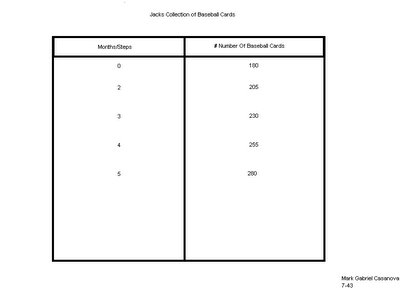b) Create an algebraic formula based upon the problem above
180 + 25(4) = 280

c) If Jack just turned 8 years old this month and Jack continues to buy the same amount of baseball cards each month, how many baseball cards will jack have when he turns 12?

Jack is going to have 1380 baseball cards when he turns 12.

Question #2: Use the chart to figure out the questions below.

Jackie is planning on having a pizza party with some of her friends. She is trying to figure out how many pizzas she has to order. She knows that each pizza has six slices of pizza.

A)If there is four people coming to the party then she will need 2 pizzas all together. Which count as 12 slices.

If the two people come and show up at the party then Jackie should order one more pizza to make three pizzas. Jackie should order 3 pizzas because look at the chart, and you will find 6 people and altogether will have 18 slices. Then you will divide and get the answer of 3 pizzas in total.

Question #3: Answer the questions below based upon this informationKathy has \$20 in her savings account. Each month she adds another \$15 to her savings account. In order to calculate how much money she will have in one year Kathy has created this algebraic formula: 20 + 15n = Savings amount
a) Calculate how much money Kathy will have in one year

Kathy is going to have \$200.00 in total.
b) Suppose Kathy counted wrong and she really has \$25 in her account instead of \$20. Change the algebraic formula to reflect this miscalculation.
If she could of made a mistake of having \$25.00 then the formula would be 25+15n= savings she would then have \$205.00 dollars.

Question #4: Solve the following equations. Show all of the steps that are needed.
A) 3n + 4n + 7 = 2n + 12 3n+4n+7=2n+12 3(1) +4(1) +7=2(1) +12 3+4+7=14
N= 1
B) 8n – (4 + 9) = 11 8n-(4+9) =11 8(3)-(4+9) =11 24-13=11
N= 3
C) (8 – 3) n + 7n + 8 = 4n + 40 (8-3) n+7n+8=4n+40 5n+7n+8=4n+40 5(4) +7(4) +8=4(4) +40
20+28+8=56
N= 4

### My Growing Post Due Tuesday May 23rd

1. (a) Jack will have the total of 280 baseball cards at the end of the next 4 months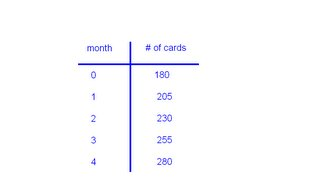(b) 180+25(C)= #of cards 180+25(4)=280 cards
First I started off multiplying the number of months, and that is 4, by 25, the number of cards he buys after each month. I then added his remaining 180 cards to get the total. Then he will have 280 cards at the end of the next 4 months.

(c) Jack will have the total of 1380 cards when he turns 12.
I got the answer by finding the difference between 8 and 12, and that is 4. I multiplied 4 by 12 and I got 48 because there are 48 months in 4 years. I then used the algebraic formula, 180+25(48). All that I did was change the variable. Then I multiplied 48 by 25 to give me 1200, and then I added the remaining 180 to get 1380 cards.

2.
(a) Jackie should order the total of 2 whole pizzas. She should order 2 pizzas because if you look at the chart and find 4 people there will be 12 slices and if there are 6 slices in each pizza. I divide 12 by 6 and that gave me 2 whole pizzas.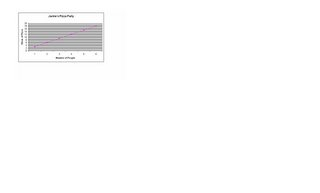(b) If two more people show up at the party, Jackie would have to order 1 more pizza, making the total 3 pizzas. She should order 3 pizzas in total becuase if you look at the chart and find 6 people there will be 18 slices. Divide 18 slices by 6 to get the total of 3 pizzas.

3. (a) Cathy will have \$200.00 dollars in her saving account. I got the answer by taking the algebraic formula of 20+15(12). I then mulitplied 12 by 15 to get 180. I then added her \$20.00 dollars to get \$200.00 dollars.
(b) If Cathy made a mistake and she had actually had \$25.00 dollars instead of \$20.00, the algebraic formula would be 25+15N= savings account total. Cathy then would have a total of \$205.00 dollars for that year.

4. (a) 3n+4n+7=2n+12

7n+7=2n+12

7n-2n(+7-7)=2n-2n(+12-7)

5n=5

n=1

(b) 8n-(4+9)=11

8n-13=11

+13 +13

8n=24

n=24/8

n=3

(c) (8-3)n+7n+8=4n+40

5n+7n+8 =4n+40

12n+8=4n+40

12n(+8-8)=4n(+40-8)

12n-4n=(4n-4n)+32

8n=32

n=4

### Kim's Growing Post

Question #1: solve all 3 parts of the problem below

Jack collects baseball cards. Jack has 180 baseball cards in his collection. At the end of each month Jack bys 25 baseball cards to add to his collection.

a) Create a T-chart showing how many basball cards Jack has at the end of the next 4 months.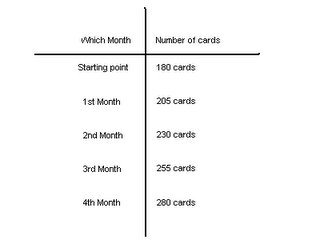b) Create an algebraic formula based upon the probelm above
180 + 25 (n) = the number of cards
n = the month

c) If Jack just turned 8 years old this month and Jack continues to buy the same amount of baseball cards each month, how many baseball cards will Jack have when he turns 12?
If you use the algebraic formula, all you have to do is: 180 + 25 (n) = the number of cards. In this case, the answer would be 1380 because you take 12 - 8 = 4. Then you take 4 (which are the years he has until he turns 12) and you times it by 12 (which is the total months in a year) and you will get 48, then if you use 48 as the variation (n), and you do the algebraic formula, you will get 1380 as your answer.

Question #2: Use the chart to figure out the questions below.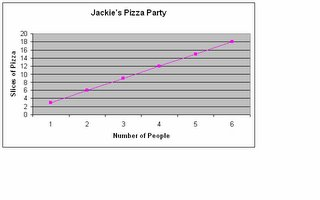Jackie is planning on having a pizza party with some of her friends. She is trying to figure out how many pizza's to order. She knows that each pizza has six slices of pizza.

a) If Jackie thinks that htere will be four people at the party (counting herself) how many pizza's should she order?
Jackie should order 2 pizza's because there are a total of 4 people (counting Jackie) and each person eats 3 slices and each pizza contains 6 slices and if you look at the chart above, you will see that 4 people need 12 slices and 12 slices means 2 pizzas in total. (this is the correction that I have made, the colour yellow)
b) If two more people show up at the party how many more pizza's does she need to order?
Jackie needs to order 3 pizza's in total because every 2 people eat up a total of 1 pizza so if there are 6 people, 6 divided by 2 is 3, Jackie will need to order 3 pizza's for her party.

Question #3: Answer the questions below based upon this information.

Kathy has \$20 in her savings account. Each month she adds another \$15 to her savings acount. In order to caculate how much money she will have in one year Kathy has created this algebraic formula: 20 + 15n = Savings amount.

a) Caculate how much money Kathy will have in one year.
I got the answer of \$200 which is the money that Kathy will have in one year. I got that answer by using Kathy's formula which is 20 + 15n (n = 12). I multiplied 15 by 12 (which are the months in a year) and I got 180. After that, I added 20 to 180 and got \$200.

b) Suppose Kathy counted wrong and she really has \$25 in her account instead of \$20. Change the algebraic formula to reflect this miscaculation.
If Kathy did counted wrong and she had \$25 in her account then all you have to do is change the number 20 to 25 and for example, it will be like this: 25 + 15n = savings amount. So Kathy will have a total of \$205 if she made the miscaculation.

Question #4: solve the following equations. Show all of the steps that are needed. ( we did not need to do these questions, so I do not have them)

Kim Thanh Vo 7-43

## Monday, May 22, 2006

### My Growing Post

Question#1

A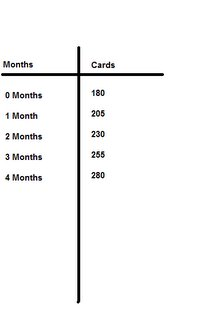C She would have 900 cards

Question#2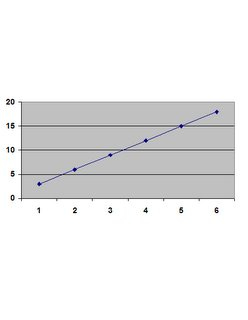A She should order 2 pizza

B She should order 1 more pizza

Question#3

A She will have \$200

B 25+15n

### My Growing Post

1.(a) Jack will have the total of 280 cards at the end of the next 4 months.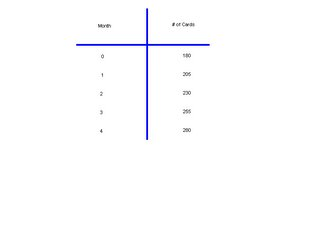(b) 180+25(C)= #of cards 180+25(4)=280 cards
First I started off by multiplying the number of months, and is 4 by 25 the number of cards he buys after each month. Then I added his remaing 180 cards to get the total. Then he will have 280 cards at the end of the next 4 months.

(c) Jack will have the total of 1380 cards when he turns 12.
I got the answer by finding out the difference between 8 and 12, and it's 4. I multiplied 4 by 12 and I got 48 because there are 48 months in 4 years. Then I used the algebraic formula, 180+25(48). All that I did was change the variable. Then I multiplied 48 by 25 to give me 1200, and then I added the remaining 180 to get 1380 cards.

2.(a) Jackie should order the total of 2 pizzas. She should order 2 pizzas because if you look at the chart and find 4 people there will be 12 slices and if there are 6 slices in each pizza I divide 12 by 6 and that gave me 2 whole pizzas.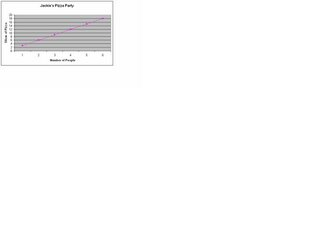(b) If two more people show up at the party Jackie should order 1 more pizza making the total of 3 pizzas. She should order 3 pizzas in total becuase if you look at the chart and find 6 people there will be 18 slices. I divided 18 slices by 6 to get the total of 3 pizzas

3.(a) Cathy will have \$200.00 dollars in her saving account. I got the answer by taking the formula of 20+15(12). I mulitplied 12 by 15 to get 180 then I added her \$20.00 dollars to get \$200.00 dollars.

(b) If Cathy made a mistake and she had actually had \$25.00 dollars instead of \$20.00 the formula would be 25+15C= savings account. Then Cathy would have a total of \$205.00 dollars for that year.

4.(a) 3n+4n+7=

2n+123(1)+4(1)+7=

2(1)+123+4+7=14

N= 1

b) 8n-(4+9)=11
8(3)-(4+9)=11
24-13=11
N= 3

(c) (8-3)n+7n+8=

4n+405n+7n+8=

4n+405(4)+7(4)+8=

4(4)+4020+28+8=56

N= 4